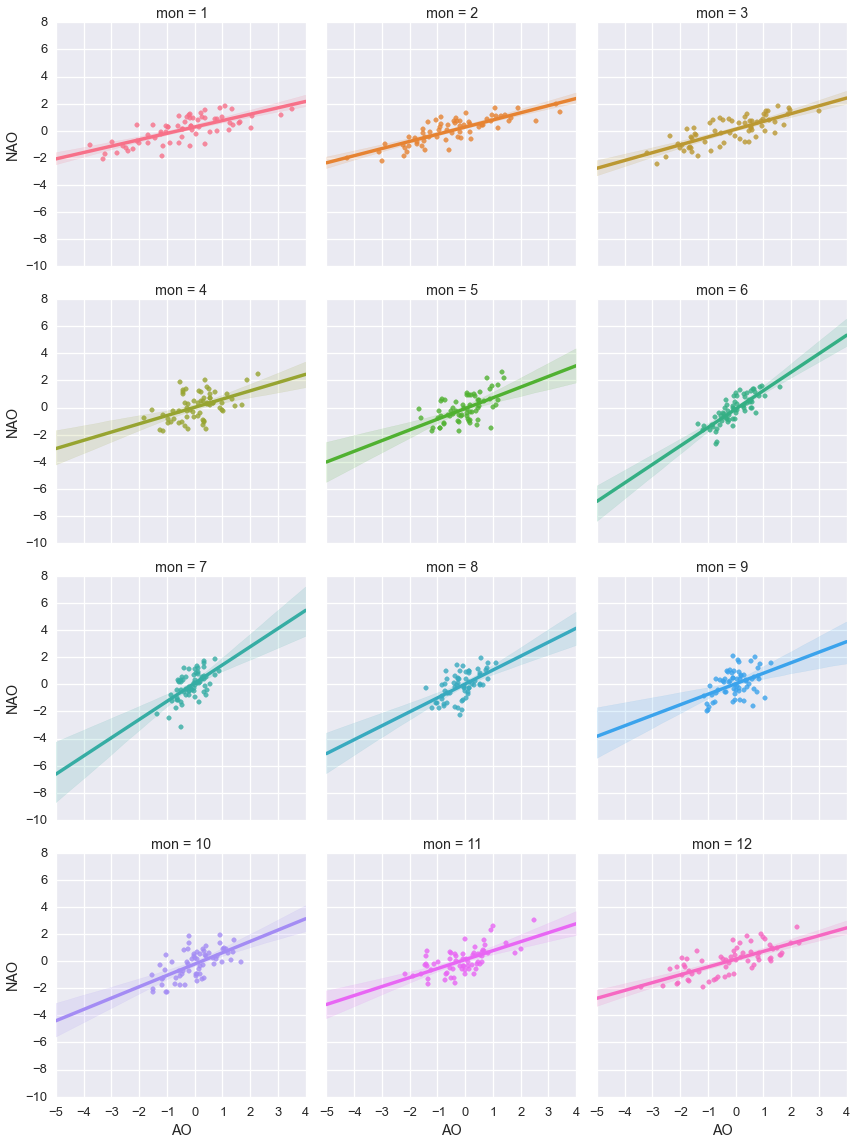# Seaborn library

Make matplotlib plots look nicer



Solution:

Seaborn

We all know and love matplotlib, but I guess most of you agree that the default output of matplotlib is ugly. One can spend hours tweaking the plots, and for sure you will get very nice result at the end - customization is one of the great powers of matplotlib after all. However there is another way - just rely on beautiful defaults created by someone else. Below I will show you couple of examples with Seaborn library, that is based on matplotlib, but make figures look much better. It also provide very simple way to draw statistical graphs, that I will also demonstrate.

Online documentation

Tutorial

In :
%matplotlib inline
import numpy as np
import pandas as pd


We going to work with climate indeces (AO, NAO, PNA indices):

In :
!wget http://www.cpc.ncep.noaa.gov/products/precip/CWlink/daily_ao_index/monthly.ao.index.b50.current.ascii


Create pandas data frame (ind) out of them:

In :
AO = pd.read_table('monthly.ao.index.b50.current.ascii', sep='\s*', \

ind = pd.DataFrame({'AO':AO, 'NAO':NAO, 'PNA':PNA})


Here is how usual plots will look like (done with pandas, but uses matplotlib under the hood):

In :
ind.plot()

Out: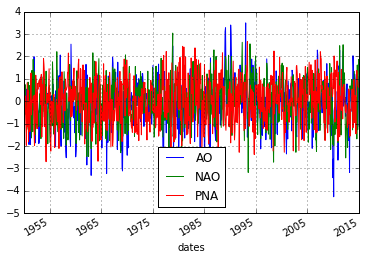In :
ind.AO.hist()

Out: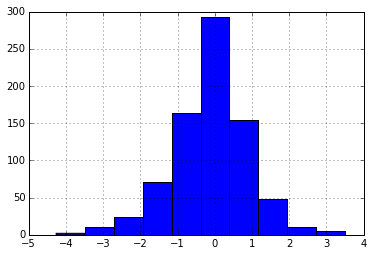They do the job - show some data, but also have certain 1990s flaivor :)

And now we just import seaborn library:

In :
import seaborn as sns


And magic happend, plots change automatically:

In :
ind.plot()

Out: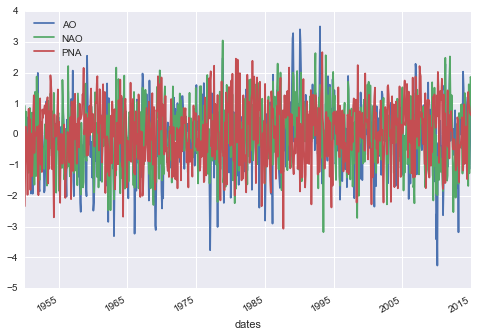In :
ind.AO.hist()

Out: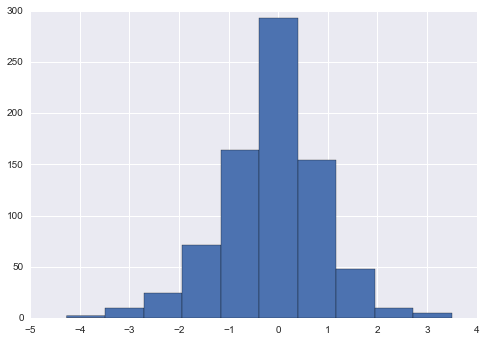Same plots, but look much better. You can tweek some basic styling:

In :
sns.set(context='talk',style='ticks')

In :
ind.AO.hist()

Out: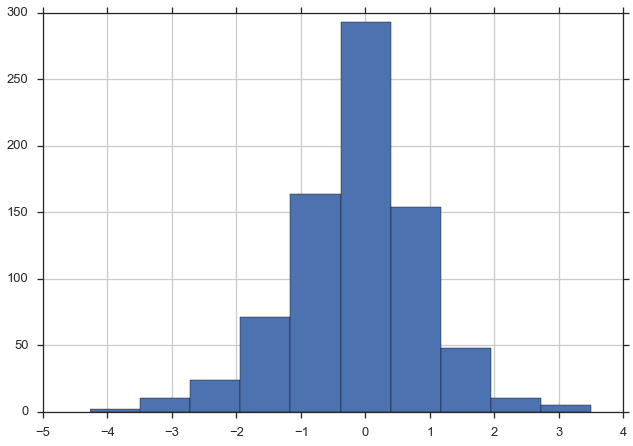In :
sns.set(context='talk',style='darkgrid')

In :
ind.AO.hist()

Out: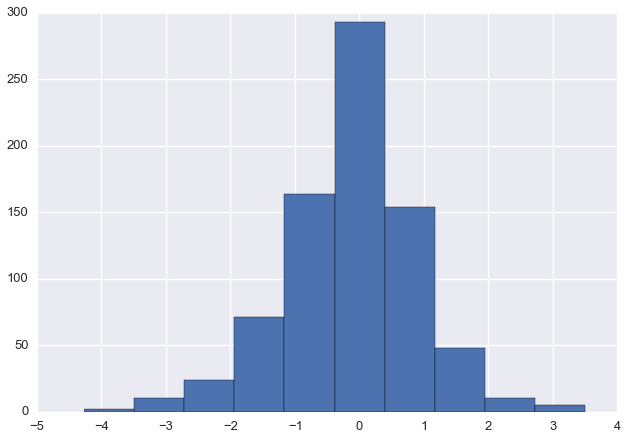But at the end you get just a usual matplotlib object, that later can be tweaked in a way you want.

## Statistical plots¶

But seaborn is mainly not about pretty plots, it's about pretty statistical plots. Regression for example:

In :
sns.regplot('AO', 'NAO', data=ind)

Out: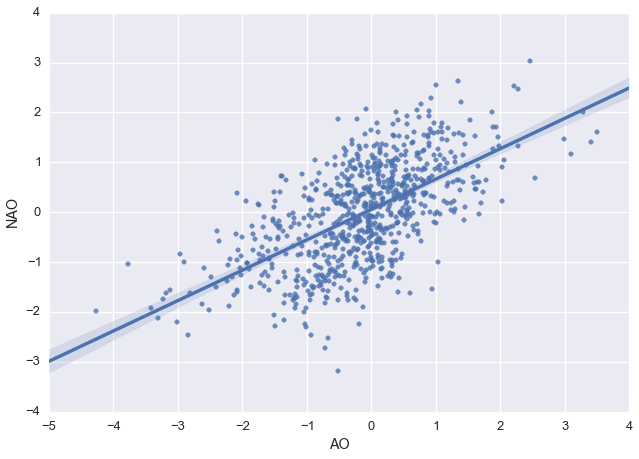In :
sns.jointplot('AO', 'NAO', data=ind)

Out: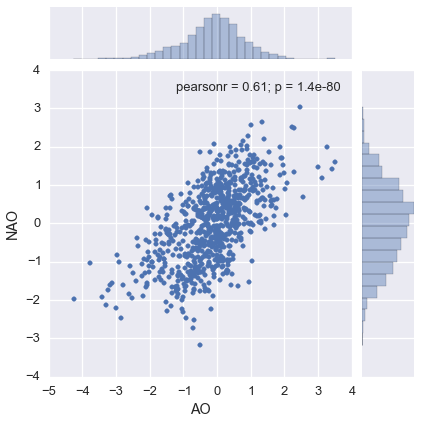In :
sns.jointplot('AO', 'NAO', data=ind, kind='reg', size=7)

Out: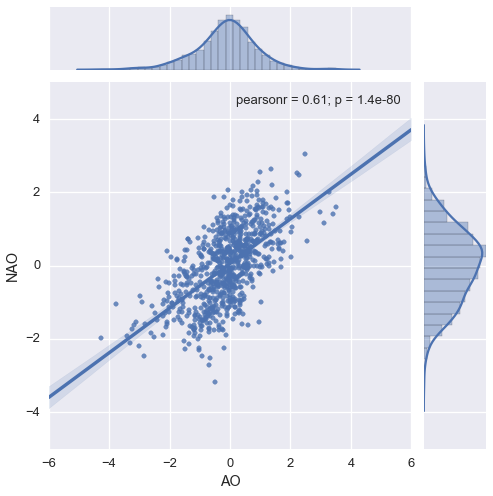Or correlation heat map:

In :
sns.corrplot(ind)

Out: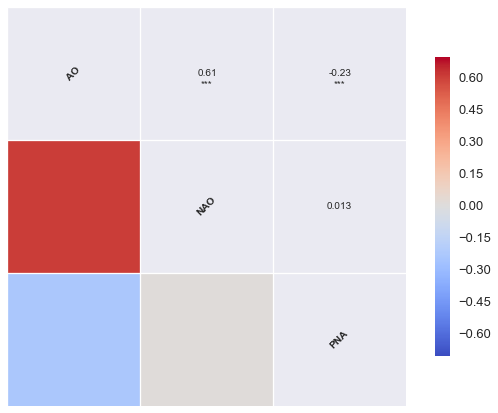This is how box plots look like with seaborn:

In :
ind['mon'] = ind.index.month

In :
sns.boxplot(ind.AO, groupby=ind.mon, alpha=0.8)

Out: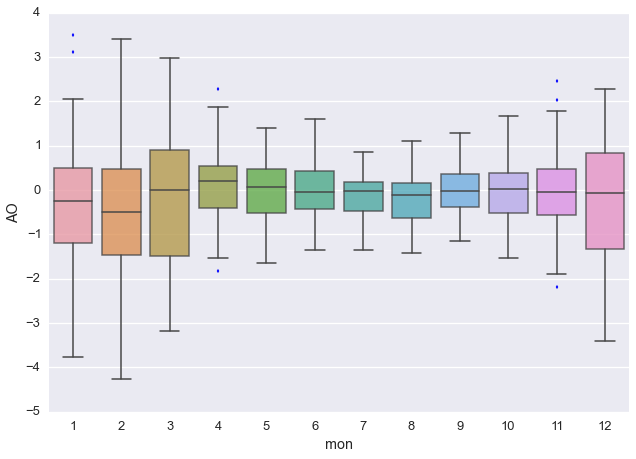In :
sns.boxplot(ind.NAO, groupby=ind.mon)

Out: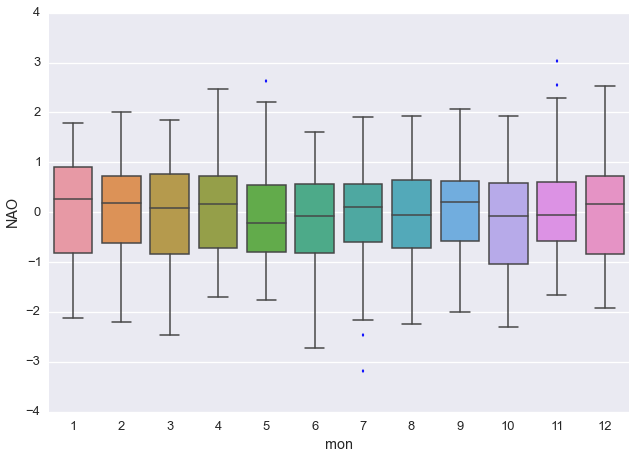In :
sns.boxplot(ind.PNA, groupby=ind.mon)

Out: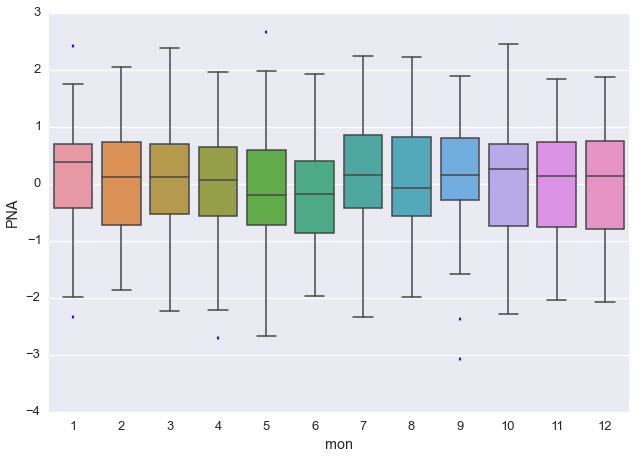There is also a violin plot:

In :
sns.violinplot(ind.NAO, groupby=ind.mon, inner="points", kernel='cos')

Out: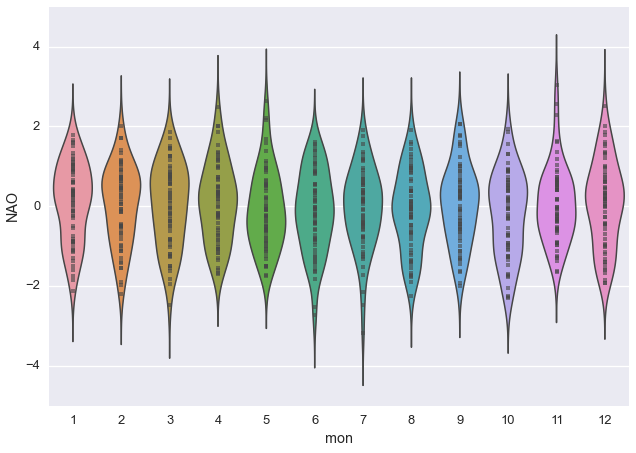Kernel density estimate:

In :
sns.kdeplot(ind.AO, shade=True)

Out: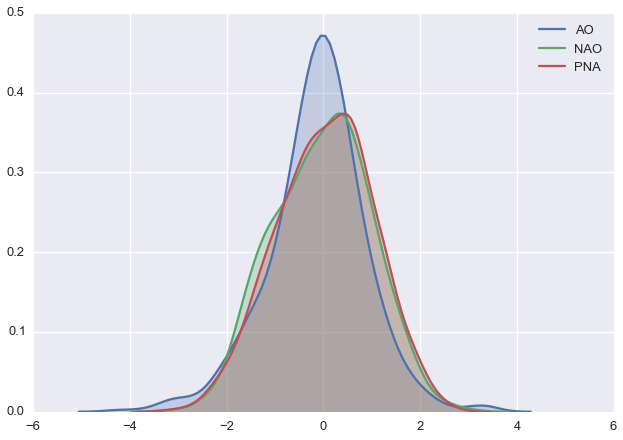Distribution:

In :
sns.distplot(ind.AO, rug=True)

Out: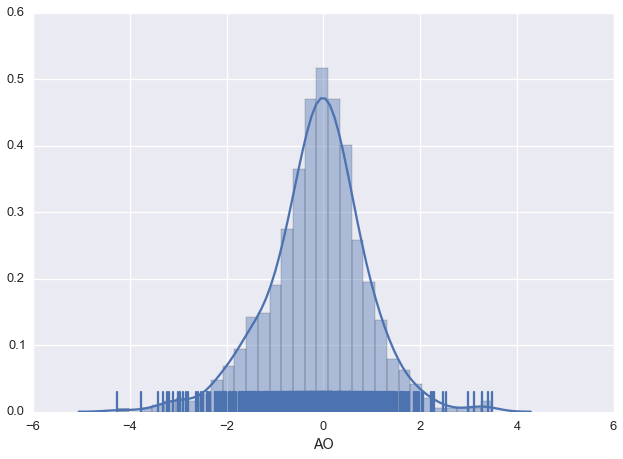Linear regression for all data together:

In :
sns.lmplot('AO', 'NAO', ind)

Out: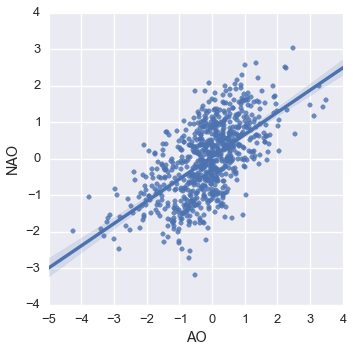For individual months (all together):

In :
sns.lmplot('AO', 'NAO', ind, hue='mon')

Out: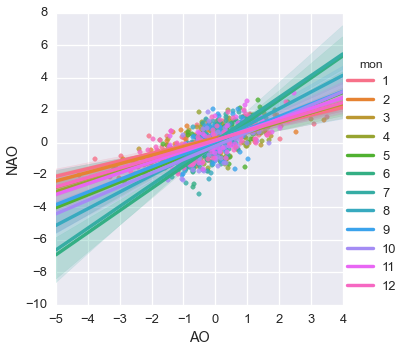For individual months one by one:

In :
sns.lmplot('AO', 'NAO', ind, hue='mon' , col='mon', col_wrap=3, size=4)

Out: